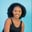Related Tags

pandas
python3

# How to use the Series.rolling() function in PandasMaria Elijah

## pandas Series.rolling() function

The pandas  Series.rolling()function offers a rolling windows function over data in the given Series.

### Syntax

Series.rolling(window, min_periods=None, center=False, win_type=None, axis=0, closed=None)


### Parameters

• window: It represents the size of the moving window.

• min_periods: It represents the minimum number of observations needed to have a value in the window. The default is None.

• center: It sets the labels of the window. The default is False. If False, the window labels are set at the right edge of the window index. Otherwise, the window labels are set at the center of the window index.

• win_type: It indicates the window type.

• axis: It can be int or string. The default value is 0. If 0 or index, the operation is performed across the rows. If 1 or columns, the operation is performed across the columns.

• closed: It is used to close the interval on the ‘right’, ‘left’, ‘both’ or ‘neither’ endpoints.

### Code example

The following code will demonstrate how to use the Series.rolling() function in pandas.

import pandas as pd

# create Series
my_series = pd.Series([17, 9, 28, 15, 23, 7, 14])

# using rolling() to compute sum()
print(my_series.rolling(2).sum())

### Code explanation

In the code above, we see the following:

• Lines 1–2: We import the pandas library.

• Line 5: We create the series, my_series.

• Line 9: We calculate the sum of data in the series over a window size of 2 using the rolling() function.

Note: A NaN was returned for the first element in the series since there is no element before it, making the sum operation impossible.

RELATED TAGS

pandas
python3

CONTRIBUTORMaria Elijah
RELATED COURSES

View all Courses

Keep Exploring

Learn in-demand tech skills in half the time